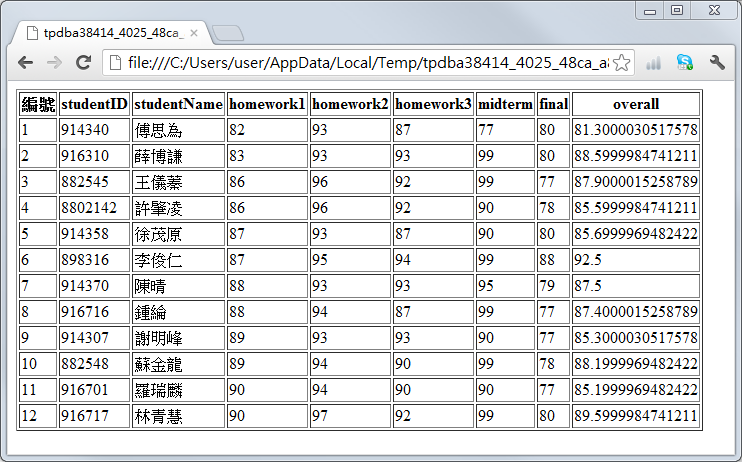## 13-5 嚙踝蕭嚙踝蕭嚙踝蕭MATLAB嚙踝蕭嚙踝蕭嚙踝蕭嚙踝蕭嚙踝蕭嚙踝蕭嚙踝蕭嚙踝蕭嚙踝蕭嚙踝蕭嚙踝蕭嚙踝蕭

INSERT INTO score (studentID, studentName, final) VALUES (''00'', ''大力水手'', 100)

Example 1: 13-與資料庫的整合/insertData01.mcopyfile('score01.mdb', 'score02.mdb'); % 將 score01.mdb 拷貝到 score02.mdb dsn = 'dsnScore02'; % 設定資料來源名稱（指到 score02.mdb） logintimeout(5); % 設定嘗試連結資料庫的時間 conn = database(dsn, '', ''); % 連結資料庫 % 設定新增資料所用的 SQL 命令 sql = 'INSERT INTO score (studentID, studentName, final) VALUES (''00'', ''大力水手'', 100)'; cursor = exec(conn, sql); % 執行 SQL 命令 % 設定查詢資料用的 SQL 命令 sql = 'select * from score'; cursor = exec(conn, sql); cursor = fetch(cursor); newScore = cursor.data % 顯示更新後 final 欄位的資料 close(cursor); % 結束 cursor 物件 close(conn); % 結束資料庫連結newScore = '914340' '傅思為'  [ 93] [ 87] [ 77] [ 80]  '916310' '薛博謙'  [ 93] [ 93] [ 99] [ 80]  '882545' '王儀蓁'  [ 96] [ 92] [ 99] [ 77]  '8802142' '許肇凌'  [ 96] [ 92] [ 90] [ 78]  '914358' '徐茂原'  [ 93] [ 87] [ 90] [ 80]  '898316' '李俊仁'  [ 95] [ 94] [ 99] [ 88]  '914370' '陳晴'  [ 93] [ 93] [ 95] [ 79]  '916716' '鍾綸'  [ 94] [ 87] [ 99] [ 77]  '914307' '謝明峰'  [ 93] [ 93] [ 90] [ 77]  '882548' '蘇金龍'  [ 94] [ 90] [ 99] [ 78]  '916701' '羅瑞麟'  [ 94] [ 90] [ 90] [ 77]  '916717' '林青慧'  [ 97] [ 92] [ 99] [ 80]  '00' '大力水手' [ 0] [NaN] [NaN] [NaN]  

UPDATE score SET final=100 WHERE final>=80

Example 2: 13-與資料庫的整合/updateData01.mcopyfile('score01.mdb', 'score02.mdb'); % 將 score01.mdb 拷貝到 score02.mdb dsn = 'dsnScore02'; % 設定資料來源名稱（指到 score02.mdb） logintimeout(5); % 設定嘗試連結資料庫的時間 conn = database(dsn, '', ''); % 連結資料庫 % 設定更新資料所用的 SQL 命令 sql = 'UPDATE score SET final=100 WHERE final>=80'; cursor = exec(conn, sql); % 執行 SQL 命令 % 設定進行資料查詢的 SQL 命令 sql = 'select studentName, final from score'; % 設定 SQL 命令 cursor = exec(conn, sql); cursor = fetch(cursor); newScore = cursor.data % 顯示更新後 final 欄位的資料 close(cursor); % 結束 cursor 物件 close(conn); % 結束資料庫連結newScore = '傅思為'  '薛博謙'  '王儀蓁' [ 77] '許肇凌' [ 78] '徐茂原'  '李俊仁'  '陳晴' [ 79] '鍾綸' [ 77] '謝明峰' [ 77] '蘇金龍' [ 78] '羅瑞麟' [ 77] '林青慧' 

DELETE FROM score WHERE final<80

Example 3: 13-與資料庫的整合/deleteData01.mcopyfile('score01.mdb', 'score02.mdb'); % 將 score01.mdb 拷貝到 score02.mdb dsn = 'dsnScore02'; % 設定資料來源名稱（指到 score02.mdb） logintimeout(5); % 設定嘗試連結資料庫的時間 conn = database(dsn, '', ''); % 連結資料庫 % 設定刪除資料所用的 SQL 命令 sql = 'DELETE FROM score WHERE final<80'; cursor = exec(conn, sql); % 設定進行資料查詢的 SQL 命令 sql = 'select studentName, final from score'; cursor = exec(conn, sql); cursor = fetch(cursor); newScore = cursor.data % 顯示更新後 final 欄位的資料 close(cursor); % 結束 cursor 物件 close(conn); % 結束資料庫連結newScore = '傅思為'  '薛博謙'  '徐茂原'  '李俊仁'  '林青慧' 

Example 4: 13-與資料庫的整合/createTable01.mcopyfile('score01.mdb', 'score02.mdb'); % 將 score01.mdb 拷貝到 score02.mdb dsn = 'dsnScore02'; % 設定資料來源名稱（指到 score02.mdb） logintimeout(5); % 設定嘗試連結資料庫的時間 conn = database(dsn, '', ''); % 連結資料庫 % 產生新資料表 friend sql = 'CREATE TABLE friend (fullName char(6), birthday date)'; exec(conn, sql); % 插入第一筆資料 sql = 'INSERT INTO friend (fullName, birthday) VALUES (''王瓊雯'', ''1983/11/03'')'; exec(conn, sql); % 插入第二筆資料 sql = 'INSERT INTO friend (fullName, birthday) VALUES (''葉佳慧'', ''1982/09/22'')'; exec(conn, sql); % 列出所有資料 cursor = exec(conn, 'select * from friend'); cursor = fetch(cursor); friend = cursor.data % 顯示更新後 friend 資料表的資料 close(cursor); % 結束 cursor 物件 close(conn); % 結束資料庫連結friend = '王瓊雯' '1983-11-03 00:00:00.0' '葉佳慧' '1982-09-22 00:00:00.0'

1. 讀入 score02.mdb 的 score 資料表
2. 計算每一個學生的期末總成績，等於作業平均乘以 30%，加上期中考成績（midterm 欄位）乘以 30%，再加上期末考（final 欄位）成績乘以 40%。
3. 將每一個學生的期末成績送到資料庫的 overall 欄位。
4. 將最後成績計算結果寫成一個網頁，以便公布給學生看。

Example 5: 13-與資料庫的整合/computeScore01.mcopyfile('score01.mdb', 'score02.mdb'); % 將 score01.mdb 拷貝到 score02.mdb dsn = 'dsnScore02'; % 設定資料來源名稱（指到 score02.mdb） logintimeout(5); % 設定嘗試連結資料庫的時間 conn = database(dsn, '', ''); % 連結資料庫 % 抓出所有的資料 cursor = exec(conn, 'select * from score'); % 執行 SQL 命令，並傳回 cursor 物件 cursor = fetch(cursor); % 經由 cursor 物件，抓取全部資料 score = cursor.data; % 將資料傳至 MATLAB 變數 score temp = columnnames(cursor); % 資料庫欄位名稱 eval(['fieldNames = {', temp, '}'';']); % 將欄位名稱指定到 fieldNames 變數 score = cell2struct(score, fieldNames, 2); % 將異質陣列 score 轉成結構陣列 % 對每一筆資料進行運算，並將結果存回資料庫 for i=1:length(score) homework=(score(i).homework1+score(i).homework2+score(i).homework3)/3; overallScore=homework*0.3+score(i).midterm*0.3+score(i).final*0.4; % 將資料寫入資料庫 sql = ['UPDATE score SET overall=', num2str(overallScore), ' where studentID=''', score(i).studentID, '''']; exec(conn, sql); end % 列出 score 資料表 cursor = exec(conn, 'select * from score'); cursor = fetch(cursor); newScore = cursor.data; % 更新後的資料 temp = columnnames(cursor); % 資料庫欄位名稱 eval(['fieldNames = {', temp, '}'';']); % 將欄位名稱指定到 fieldNames 變數 newScore = cell2struct(newScore, fieldNames, 2);% 將異質陣列 newScore 轉成結構陣列 close(cursor); % 結束 cursor 物件 close(conn); % 結束資料庫連結 struct2html(newScore); % 顯示結構陣列 newScore 於瀏覽器MATLAB程式設計：進階篇